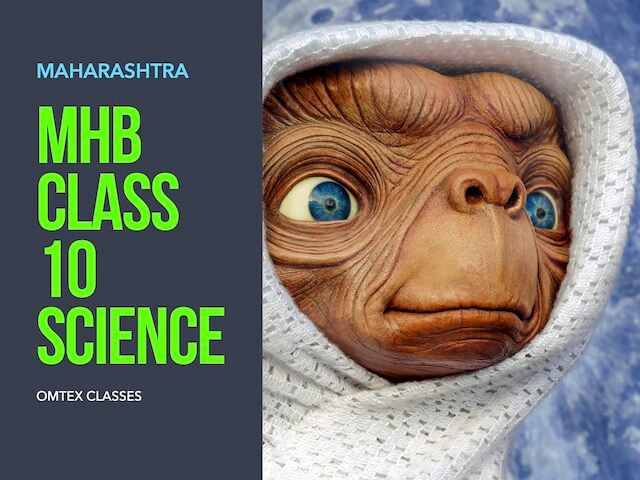SSC BOARD PAPERS IMPORTANT TOPICS COVERED FOR BOARD EXAM 2024

### Question 10. Solve the following example. The radius of planet A is half the radius of planet B. If the mass of A is MA, what must be the mass of B so that the value of g on B is half that of its value on A?

Question 10.

Solve the following example.
The radius of planet A is half the radius of planet B. If the mass of A is MA, what must be the mass of B so that the value of g on B is half that of its value on A?

We know that acceleration due to gravity (g) is given by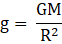Where G = universal gravitational constant;

M = mass of the planet;

R = radius of the planet;

Mass of Planet A = MA;

Radius of Planet A = RA;

Value of ‘g’ on A = gA;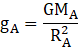Mass of Planet B = MB;

Radius of Planet B = RB;

Value of ‘g’ on B = gB;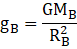Also it is given that;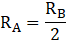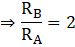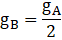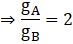Therefore;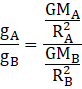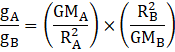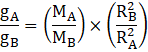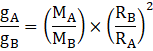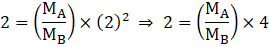(from question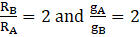)

⇒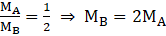Therefore the mass of planet B should be two times the mass of planet A i.e MB = 2MA .